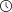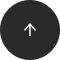- 9 minutes read

# 18 Useful Important SQL Functions to Learn ASAP

### Beginning with SQL: Useful Functions to Master

Learning a new programming language can seem intimidating. Like any other language, a programming language has a large vocabulary that you need to master. In this article, we'll look at some of the most useful SQL functions that you need to know.

There are many useful SQL functions for working with different data types. Once you practice with some of the most common ones, you'll gain enough confidence to move on to more advanced material. So, let's examine some of these most common SQL functions in greater detail.

### 1. STRING FUNCTIONS

A string is just a series of one or more characters that have been strung together. Quite simply, string functions deal with strings!

#### Length

The most simple SQL string function is length. As its name suggests, `length` returns the number of characters that a given string contains (including spaces and punctuation). Let's look at a simple PostgreSQL select statement and feed it with the string 'Hello, my name is':

```select length('Hello, my name is');
```

The result of running this query is 17. If you manually count the number of characters, spaces included, in the above string, you'll find that 17 is a precise match! While `length` may at first seem like a trivial function, you will actually use it very frequently.

#### Trim

Let's trim some fat! Another example from the most useful SQL functions category is `trim`. It eliminates excess spaces and tabs from the beginning and end of a string that we pass in as its argument. For instance, if we pass in the string ' Hello, my name is ', we will receive the same string but without all those leading and trailing spaces. Here's a basic query with that string:

```select length('  Hello, my name is  ');
```

As expected, the above query returns `'Hello, my name is'`.

#### Concat

The `concat` function combines (concatenates) two or more strings that we pass in as its arguments. Here's an example of string concatenation in action:

```select concat(' Hello, ', 'my name is ', 'Paul');
```

This query returns the string `'Hello, my name is Paul'`.

#### Upper

Given a string, upper returns the same string but with all its characters cast to uppercase. So, if you'd like everyone to hear you loud and clear, you can write the following query:

```select upper('Hello, my name is Paul');
```

This returns the string `'HELLO, MY NAME IS PAUL'`.

#### Lower

The function `lower` is the direct opposite of `upper` — it takes a string and returns the same string with all its characters cast to lowercase. Here's a query that uses lower:

```select lower('Hello, my name is Paul');
```

Predictably, we get the string `'hello, my name is paul'`.

#### Initcap

This function capitalizes the first (initial) letter of each word in a given string. For example, you can use `initcap` to ensure that the name someone has provided adheres to proper capitalization rules. Here's an example of the function in use:

```select initcap('paul anderson');
```

The result of the above query is `'Paul Anderson'`.

Of course, there are many more useful SQL functions used for strings, like `replace`, `substr`, and others. To learn more about these, check out LearnSQL.com's Standard SQL Functions interactive course! Thanks to it, you'll learn about other common SQL functions I didn't mention in this article.

### 2. NUMERICAL FUNCTIONS

Numerical functions simply work with numbers, and there are quite a lot of useful SQL functions in this category. Let's discuss a few of these.

#### Abs

Trimming? Abs? Yeah, I know what you're thinking, but this isn't a workout program. Abs is shorthand for absolute; it's one of the most common SQL functions that calculates the absolute value of a numeric value we pass in as its argument. In other words, abs returns the positive version of a given number. Here's an example:

```select abs(-22),abs(22);
```

If you've been paying attention in math class, you'll know that both of these calls to abs return the number 22.

#### Round

The `round` function takes a floating-point (decimal) number and rounds it just like you normally would on paper. Take a look at this simple example:

```select round(5.4),round(5.5),round(5.6);
```

We would get 5 (next smallest integer), 6 (next largest integer), and 6 (next largest integer), respectively, for the above three calls to round.

#### Ceil

The `ceil` function returns the ceiling of a number—the first integer greater than or equal to that number. To visualize this process, place the number in question (say -0.5) on a standard number line and move left to right, searching for the next largest integer (in this case, that's 0). Here's an additional example:

```select ceil(5.4),ceil(5.5),ceil(6);
```

We receive the numbers 6, 6, and 6 for these three calls.

#### Floor

`Floor` is the reverse of the `ceil` function; it returns the floor of the decimal number we pass in as its argument — the first integer that is less than or equal to that number. For example, the floor of -0.5 is -1, as that is the first integer that is less than -0.5. With the number line visualization, you start at the given number and move right to left in search of an integer. If we repeat the above calls with the `floor` function instead:

```select floor(5.4),floor(5.5),floor(6);
```

We receive these three numbers after running the above query: 5, 5, and 6.

#### Sign

One of the simpler functions on this list, sign takes a number as its argument and returns -1 if the number is negative, 0 if the number is 0, and 1 if the number is positive. It's as easy as that! Here's an example:

```select sign(-5),sign(0),sign(5);
```

This query returns the following numbers: -1, 0, and 1.

#### Mod

Mod stands for modulo. It is a very powerful yet still one of the most common SQL functions that returns the remainder of the first argument divided by the second argument. For example, dividing 5 by 2 results in a remainder of 1. Thus, the function call `mod(5, 2)` returns the number 1!

The modulo operator has many useful applications, and one of its common uses is determining whether a given number is even or odd. Recall that if a number is even, the remainder of dividing that number by 2 is 0. Otherwise, the remainder is 1. Thus, given a number n, you can perform `mod(n, 2)` to determine whether n is even or odd!

There are many more useful SQL functions in the numerical category you should know, such as `trunc`, `trigonometric`, `logarithmic`, `power`, `root`, and more. The best place to master these is LearnSQL.com's Standard SQL Functions course. If you haven't already, go ahead and check it out!

### 3. DATE FUNCTIONS

As this category's name suggests, date functions work with dates! In this section, we'll take a look at some of the most useful SQL functions of this type.

#### Current_date

First on our list is `current_date`, an extremely useful SQL function that … returns the current date! Here's an example query:

```select current_date;
```

For us, this will return 2018-01-04.

#### Extract

This common SQL function allows you to extract certain parts of a date (such as the day, month, or year) that you pass in as an argument. Here's an example of all three uses:

```select extract(day from date'2017-1-1'),extract(month from date'2017-1-1'),extract(year from date'2017-1-1');
```

The above query returns the following three numbers: 1, 1, and 2017.

#### Date_trunc

The `date_trunc` function truncates the values of a given date to a specific granularity. Let's say you have a date, but you want to have only the first day of the month of that date you would truncate the date value to a 'month' parameter. Let's look at an example:

```select date_trunc('month',date'2017-4-2')
```

This would return the date of 04-01-2017. If we would feed the year value as a granularity:

```select date_trunc('year',date'2017-4-2')
```

We would receive the first day of the inputted year, 01-01-2017.

### 4. MISCELLANEOUS FUNCTIONS

In addition to the functions we've covered so far, there are a couple miscellaneous functions that are commonly used in SQL programming.

#### Coalesce

Sometimes, you'll come across null values when working with tabular data. These represent absent or missing information. Unfortunately, any calculation involving a null value will return null, and this is not always ideal.

The `COALESCE` function takes a list of arguments and returns the first of these that does not contain a value of null. In other words, if SQL finds that the first argument you provided is null, it will move on to evaluate the second argument, repeating the process until it either finds one that isn't null or simply runs out of arguments.

For example, suppose we're working with a table that has a numeric column named potentially_null_column. Let's say this column has several values—some that are null and others that are not. Suppose we execute the following code:

```select COALESCE(potentially_null_column, 0);
```

Here, the query will use a value of 0 whenever a cell in the potentially_null_column stores a value of null. For cells in that column that are not null, the query will simply use their values. This SQL function is most useful in calculations involving columns that may contain null values, as it prevents unexpected behavior. To learn more about `COALESCE` and its uses, check out this useful article.

#### Greatest

Another one from the most useful SQL functions, greatest, takes a list of expressions and returns the largest of them. Here's an example of it being used:

```select greatest(1,2,3,5,6);
```

Naturally, this query returns 6, as that is the greatest value in the list we provided.

#### Least

Least is the direct inverse of greatest. If we write the following query:

```select least(1,2,3,5,6);
```

This query returns 1, as that is the smallest value in the list we provided.

### And Once You Know These Useful SQL Functions...

There is a variety of useful SQL functions for manipulating strings, numbers, dates, and other data types. Now that you've learned a bit about the basics, you should consider delving deeper into these subjects to expand on what you already know. For that, there's simply no better place to start than LearnSQL.com! Our  Advanced SQL Track course pack includes the following SQL courses:

1. Standard SQL Functions – in this course you'll learn how to process numerical, text, and other types of data with most useful SQL functions.
2. Window Functions – here you’ll learn windowing functions also known as analytic SQL functions.
3. Recursive Queries – in this course you’ll learn how to process trees and graphs in SQL, and how to effectively organize long SQL queries and subqueries.

You've already learned the most common SQL functions. Now, take your self-development to the next level and master the most challenging SQL queries!